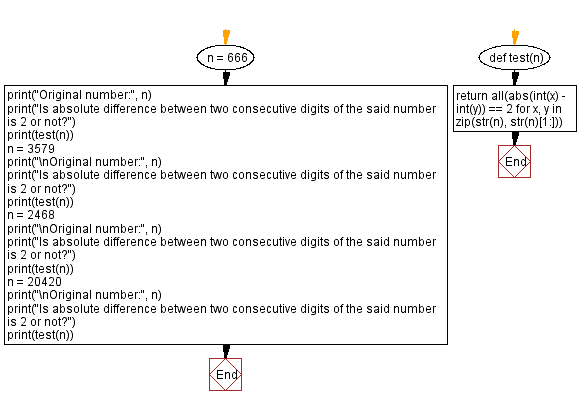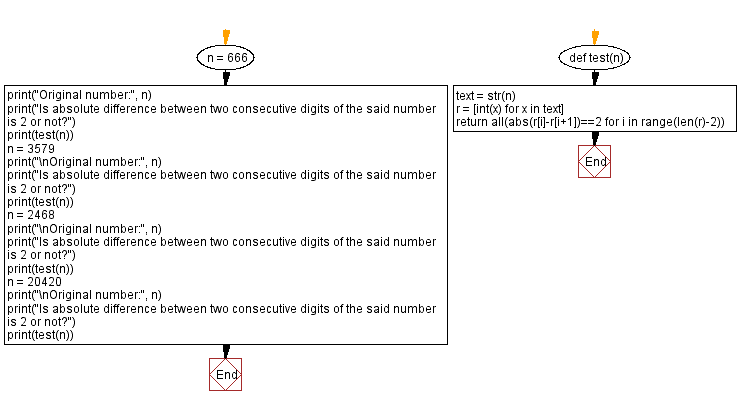﻿ Python - Absolute difference between two consecutive digits# Python Exercises: Absolute difference between two consecutive digits

## Python Math: Exercise-92 with Solution

Write a Python program to check the absolute difference between two consecutive digits is two or not. Return true otherwise false.

Sample Data:
(666) -> False
(3579) -> True
(2468) -> True
(20420) -> False

Sample Solution-1:

Python Code:

``````def test(n):
return all(abs(int(x) - int(y)) == 2 for x, y in zip(str(n), str(n)[1:]))

n = 666
print("Original number:", n)
print("Is absolute difference between two consecutive digits of the said number is 2 or not?")
print(test(n))
n = 3579
print("\nOriginal number:", n)
print("Is absolute difference between two consecutive digits of the said number is 2 or not?")
print(test(n))
n = 2468
print("\nOriginal number:", n)
print("Is absolute difference between two consecutive digits of the said number is 2 or not?")
print(test(n))
n = 20420
print("\nOriginal number:", n)
print("Is absolute difference between two consecutive digits of the said number is 2 or not?")
print(test(n))
```
```

Sample Output:

```Original number: 666
Is absolute difference between two consecutive digits of the said number is 2 or not?
False

Original number: 3579
Is absolute difference between two consecutive digits of the said number is 2 or not?
True

Original number: 2468
Is absolute difference between two consecutive digits of the said number is 2 or not?
True

Original number: 20420
Is absolute difference between two consecutive digits of the said number is 2 or not?
False
```

Flowchart:## Visualize Python code execution:

The following tool visualize what the computer is doing step-by-step as it executes the said program:

Sample Solution-2:

Python Code:

``````def test(n):
text = str(n)
r = [int(x) for x in text]
return all(abs(r[i]-r[i+1])==2 for i in range(len(r)-2))

n = 666
print("Original number:", n)
print("Is absolute difference between two consecutive digits of the said number is 2 or not?")
print(test(n))
n = 3579
print("\nOriginal number:", n)
print("Is absolute difference between two consecutive digits of the said number is 2 or not?")
print(test(n))
n = 2468
print("\nOriginal number:", n)
print("Is absolute difference between two consecutive digits of the said number is 2 or not?")
print(test(n))
n = 20420
print("\nOriginal number:", n)
print("Is absolute difference between two consecutive digits of the said number is 2 or not?")
print(test(n))
```
```

Sample Output:

```Original number: 666
Is absolute difference between two consecutive digits of the said number is 2 or not?
False

Original number: 3579
Is absolute difference between two consecutive digits of the said number is 2 or not?
True

Original number: 2468
Is absolute difference between two consecutive digits of the said number is 2 or not?
True

Original number: 20420
Is absolute difference between two consecutive digits of the said number is 2 or not?
False
```

Flowchart:## Visualize Python code execution:

The following tool visualize what the computer is doing step-by-step as it executes the said program:

Python Code Editor:

Have another way to solve this solution? Contribute your code (and comments) through Disqus.

Previous Python Exercise: Next number containing only distinct digits.
Next Python Exercise: Rearrange the digits of a number.

What is the difficulty level of this exercise?

Test your Programming skills with w3resource's quiz.

﻿

## Python: Tips of the Day

Getting sorted iterable (can sort by "compare" function):

```>>> a = [1, 2, -3]
>>> sorted(a)
[-3, 1, 2]

>>> sorted(a,key=abs)
[1, 2, -3]
```